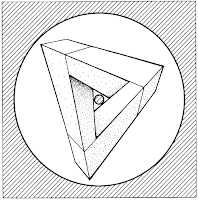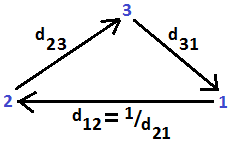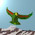## Sunday, February 6, 2011

### Cohomology Revisited

Modern physicists and mathematicians like to draw diagrams with strings and ribbons. An object in a category is often depicted by a strand with an arrow (as in (a)). Recognising that an arrow represents two colours, we might like to use three colours instead, as in (b). A tensor product of objects is given by placing their strands side by side. In (c) we thicken the strand to a ribbon, which allows twists in our diagram networks. But why stop there? We might as well use the three dimensions at our disposal and thicken the ribbon backwards, as in (d). Finally, taking multiple coloured beams, we can form braided networks from them.One of the simplest diagrams in a $1$-dimensional category is a triangle. With beams we could draw this:This is Penrose's triangle. He uses it to discuss the first cohomology group, where the numbers are interpreted as positive distances. Elements of a cohomology group are classes of cocycles, and in one dimension a category theorist would draw the cocycle condition as a trianglewhere the $d_{ij}$ are distances of observation. We have a coboundary when the cycle composition results in the number $1$, where an inverted arrow corresponds to taking the reciprocal positive number. That is, each $d_{ij}$ is a ratio $x_{i}/ x_{j}$. Reciprocation is the multiplicative analog of a minus sign for addition. In a higher dimensional category, more complicated polytopes are used to specify cocycle conditions. When these polytopes are canonical axioms for a suitable class of categories, the cohomology should be universal.

1.By the way, Happy New Year everyone! And best wishes for Waitangi Day.

2.The same. Rabbit year should be a good one.

This Penrose triangle is a helix, that is a soliton. It is clearly seen in his other 'impossible figures' from your link.

I think this is important to remember. From a soliton you can also get the quasiparticles, as a dimuon.

Note: Only a member of this blog may post a comment.